×#### Thank you for registering.

One of our academic counsellors will contact you within 1 working day.

Click to Chat

1800-1023-196

+91-120-4616500

CART 0

• 0

MY CART (5)

Use Coupon: CART20 and get 20% off on all online Study Material

ITEM
DETAILS
MRP
DISCOUNT
FINAL PRICE
Total Price: Rs.

There are no items in this cart.
Continue Shopping• Complete JEE Main/Advanced Course and Test Series
• OFFERED PRICE: Rs. 15,900
• View Details

```Chapter 1: Real Numbers Exercise – 1.6

Question: 1

Without actually performing the long division, state whether the following rational numbers will have a terminating decimal expansion or a non-terminating repeating decimal expansion.

(i)  23/8

(ii) 125/441

(iii) 35/50

(iv) 77/210Solution:

(i) The given number is 23/8

Here, 8 = 23 and 2 is not a factor of 23.

So, the given number is in its simplest form.

Now, 8 = 23 is of the form 2m × 5n, where m = 3 and n = 0.

So, the given number has a terminating decimal expansion.

(ii) The given number is 125/441

Here, 441 = 32 × 72 and none of 3 and 7 is a factor of 125.

So, the given number is in its simplest form.

Now, 441 = 32 × 72 is not of the form 2m × 5n

So, the given number has a non-terminating repeating decimal expansion.

(iii) The given number is 35/50 and HCF (35, 50) = 5.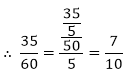Here, 7/10 is in its simplest form.

Now, 10 = 2 × 5 is of the form 2m × 5n, where in = 1 and n = 1.

So, the given number has a terminating decimal expansion.

(iv) The given number is 77/210 and HCF (77, 210) = 7.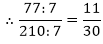Here, 11/30 is in its simplest form. 30

Now, 30 = 2 × 3 × 5 is not of the form 2m × 5n.

So, the given number has a non-terminating repeating decimal expansion.

(v) The given number is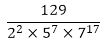Clearly, none of 2, 5 and 7 is a factor of 129.

So, the given number is in its simplest form.

Question: 2

Write down the decimal expansions of the following rational numbers by writing their denominators in the form of 2m × 5n, where m, and n, are the non- negative integers.

(i)  3/8

(ii)  13/125

(iii)  7/80

(iv) 14588/625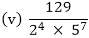Solution:

(i) The given number is 3/8

Clearly, 8 = 23 is of the form 2m × 5n, where m = 3 and n = 0.

So, the given number has terminating decimal expansion.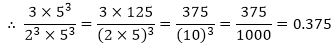(ii) The given number is 13/125

Clearly, 125 = 53 is of the form 2m × 5″, where m = 0 and n = 3.

So, the given number has terminating decimal expansion.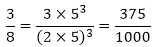(iii) The given number is 7/80.

Clearly, 80 = 24 × 5 is of the form 2m × 5n, where m = 4 and n = 1.

So, the given number has terminating decimal expansion.(iv) The given number is 14588/625

Clearly, 625 = 54 is of the form 2m × 5n, where m = 0 and n = 4.

So, the given number has terminating decimal expansion?(v) The given number is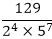Clearly, 22 × 57 is of the form 2m × 5n, where in = 2 and n = 7.

So, the given number has terminating decimal expansion?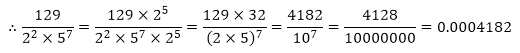Question: 3

What can you say about the prime factorization of the denominators of the following rational?

(i) 43.123456789(iv) 0.120120012000120000

Solution:

(i) Since 43.123456789 has terminating decimal expansion. So, its denominator is of the form 2m × 5n, where m, n are non-negative integers.

(ii) Since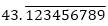has non-terminating decimal expansion. So, its denominator has factors other than 2 or 5.

(iii) Since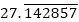has non-terminating decimal expansion. So, its denominator has factors other than 2 or 5.

(iv) Since 0.120120012000120000 … has non-terminating decimal expansion. So, its denominator has factors other than 2 or 5.
```### Course Features

• 728 Video Lectures
• Revision Notes
• Previous Year Papers
• Mind Map
• Study Planner
• NCERT Solutions
• Discussion Forum
• Test paper with Video Solution